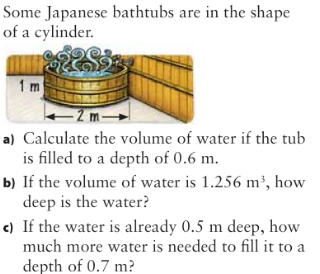## Tuesday, March 15, 2011

### Arween's Volume Post

Cylinder Volume and Volume Problems
7.3a)
R=D ÷ 2
R=2 ÷ 2
R=1m

V=π • r • r • h
V=(3.14•1•1)X 0.6
V=1.884m3
The volume of the tub with a depth of 0.6m is 1.884m3

b)
To find the height of the tub divide the Volume to the Area of the Base. Before that find the radius first.

R=D ÷ 2
R=2 ÷ 2
R=1m
Then find the area of the circle.

A=π * r * r
A=3.14 * 1 * 1
A=3.14m
Then Divide the Volume to the area of the circle.
H=1.256m3 ÷ 3.14m
H=0.4m

c)
Step 1. Find the volume of the tub with a depth of 0.5m
Step 2. Find the volume of the tub with a depth of 0.7m
step 3. Subtract the volume of the the tub with a depth of 0.7m to the tub with 0.5m.
Like this......
R=D ÷ 2
R= 2 ÷ 2
R=1m

Tub with a depth of 0.5m:
V=π*r*r*h
V=(3.14*1*1) * 0.5
V=1.57m3

Tub with a depth of 0.7m
V=π*r*r*h
V=(3.14*1*1) * 0.7
V=2.198m3

Then Subtract.
2.198m3 - 1.57m3
=0.628m3

it would take 0.628m3 to fill the tub with water at 0.7m.

Outside:
R=D ÷ 2
R=1 ÷ 2
R=0.5m

V=π * r * r * h
V=(3.14*0.5*0.5) * 10
V=7.85m3

Inside:
R= D ÷ 2
R= 0.8 ÷ 2
R=0.4m

V=π * r * r * h
V=(3.14*0.4*0.4) * 10
V=5.024m3

Now Subtract the outside diameter to the inside diameter.
7.85m3 - 5.024m3
=2.8m3